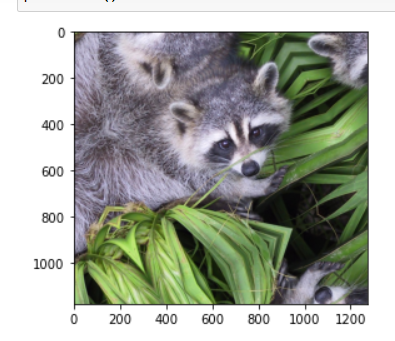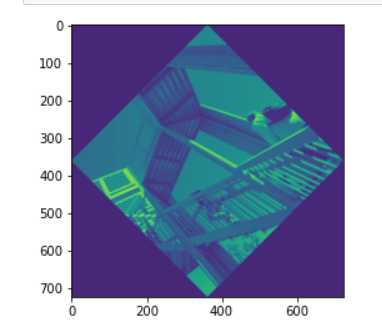Related Articles

# Rotate a picture using ndimage.rotate Scipy

• Last Updated : 03 Jan, 2021

Prerequisites: Mathplotlib, Scipy

Some of the most common tasks in image processing are displaying images, Basic manipulations, Image filtering, Image segmentation.
In this article, we will use a SciPy module “ndimage.rotate()” to rotate. The SciPy ndimage submodule is dedicated to image processing. Here, ndimage means an n-dimensional image.

Approach:

• Import all the required modules.
• SciPy comes with some images, We use those images.
• Call and pass Parameter in ndimage.rotate( ).
• Display Image.

Step 1: Import module.

## Python3

 `from` `scipy ``import` `ndimage, misc``from` `matplotlib ``import` `pyplot as plt`

Step 2: The misc package in SciPy comes with some images. We use those images to learn the image manipulations.

## Python3

 `from` `scipy ``import` `ndimage, misc``from` `matplotlib ``import` `pyplot as plt`` ` `panda ``=` `misc.face()`

Step 3: The SciPy “ndimage” submodule is dedicated to image processing. Here, “ndimage” means an n-dimensional image.

Syntax:

scipy.ndimage.rotate(input, angle)

Parameter:

• input: The input array.
• angle: The rotation angle in degrees.
• mode: {‘reflect’, ‘constant’, ‘nearest’, ‘mirror’, ‘wrap’}, optional

Returns: The rotated input.

## Python3

 `from` `scipy ``import` `ndimage, misc``from` `matplotlib ``import` `pyplot as plt``panda ``=` `misc.face()`` ` `#image rotated 135 degree``panda_rotate ``=` `ndimage.rotate(panda, ``135``, mode ``=` `'constant'``)`

Below is the Implementation:

Example 1:

## Python3

 `from` `scipy ``import` `ndimage, misc``from` `matplotlib ``import` `pyplot as plt`` ` `panda ``=` `misc.face()``#image rotated 35 degree``panda_rotate ``=` `ndimage.rotate(panda, ``35``,``                              ``mode ``=` `'mirror'``)``plt.imshow(panda_rotate)``plt.show()`

Output:Example 2:

## Python3

 `from` `scipy ``import` `ndimage, misc``from` `matplotlib ``import` `pyplot as plt`` ` `panda ``=` `misc.ascent()`` ` `#image rotated 360 degree``panda_rotate ``=` `ndimage.rotate(panda, ``45``, ``                              ``mode ``=` `'constant'``)``plt.imshow(panda_rotate)``plt.show()`

Output:Attention geek! Strengthen your foundations with the Python Programming Foundation Course and learn the basics.

To begin with, your interview preparations Enhance your Data Structures concepts with the Python DS Course. And to begin with your Machine Learning Journey, join the Machine Learning – Basic Level Course

My Personal Notes arrow_drop_up